<![CDATA[Journal of Applied Probability]]>https://www.cambridge.org/core/journals/journal-of-applied-probability/latest-issueJournal of Applied Probability0021900214756072Cambridge University Press<![CDATA[Giant descendant trees, matchings, and independent sets in age-biased attachment graphs]]>

We study two models of an age-biased graph process: the-version of the preferential attachment graph model (PAM) and the uniform attachment graph model (UAM), with m attachments for each of the incoming vertices. We show that almost surely the scaled size of a breadth-first (descendant) tree rooted at a fixed vertex converges, for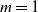, to a limit whose distribution is a mixture of two beta distributions and a single beta distribution respectively, and that for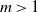the limit is 1. We also analyze the likely performance of two greedy (online) algorithms, for a large matching set and a large independent set, and determine – for each model and each greedy algorithm – both a limiting fraction of vertices involved and an almost sure convergence rate.

]]>
https://dx.doi.org/10.1017/jpr.2021.59?rft_dat=source%3Ddrssdoi:10.1017/jpr.2021.59Giant descendant trees, matchings, and independent sets in age-biased attachment graphs29932410.1017/jpr.2021.59https://dx.doi.org/10.1017/jpr.2021.59?rft_dat=source%3Ddrss2022-04-25Journal of Applied Probability2022-04-25Acan, HüseyinFrieze, AlanPittel, Boris
<![CDATA[Log-concavity and other concepts of bivariate increasing failure rate distributions]]>

Log-concavity of a joint survival function is proposed as a model for bivariate increasing failure rate (BIFR) distributions. Its connections with or distinctness from other notions of BIFR are discussed. A necessary and sufficient condition for a bivariate survival function to be log-concave (BIFR-LCC) is given that elucidates the impact of dependence between lifetimes on ageing. Illustrative examples are provided to explain BIFR-LCC for both positive and negative dependence.

]]>
https://dx.doi.org/10.1017/jpr.2021.54?rft_dat=source%3Ddrssdoi:10.1017/jpr.2021.54Log-concavity and other concepts of bivariate increasing failure rate distributions32533710.1017/jpr.2021.54https://dx.doi.org/10.1017/jpr.2021.54?rft_dat=source%3Ddrss2022-02-08Journal of Applied Probability2022-02-08Gupta, Ramesh C.Kirmani, S. N. U. A.
<![CDATA[Disorder detection with costly observations]]>

We study the Wiener disorder detection problem where each observation is associated with a positive cost. In this setting, a strategy is a pair consisting of a sequence of observation times and a stopping time corresponding to the declaration of disorder. We characterize the minimal cost of the disorder problem with costly observations as the unique fixed point of a certain jump operator, and we determine the optimal strategy.

]]>
<![CDATA[How a probabilistic analogue of the mean value theorem yields stein-type covariance identities]]>

A method for the construction of Stein-type covariance identities for a nonnegative continuous random variable is proposed, using a probabilistic analogue of the mean value theorem and weighted distributions. A generalized covariance identity is obtained, and applications focused on actuarial and financial science are provided. Some characterization results for gamma and Pareto distributions are also given. Identities for risk measures which have a covariance representation are obtained; these measures are connected with the Bonferroni, De Vergottini, Gini, and Wang indices. Moreover, under some assumptions, an identity for the variance of a function of a random variable is derived, and its performance is discussed with respect to well-known upper and lower bounds.

]]>
https://dx.doi.org/10.1017/jpr.2021.61?rft_dat=source%3Ddrssdoi:10.1017/jpr.2021.61How a probabilistic analogue of the mean value theorem yields stein-type covariance identities35036510.1017/jpr.2021.61https://dx.doi.org/10.1017/jpr.2021.61?rft_dat=source%3Ddrss2022-01-18Journal of Applied Probability2022-01-18Psarrakos, Georgios
<![CDATA[Efficient conditional Monte Carlo simulations for the exponential integrals of Gaussian random fields]]>

We consider a continuous Gaussian random field living on a compact set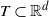. We are interested in designing an asymptotically efficient estimator of the probability that the integral of the exponential of the Gaussian process over T exceeds a large threshold u. We propose an Asmussen–Kroese conditional Monte Carlo type estimator and discuss its asymptotic properties according to the assumptions on the first and second moments of the Gaussian random field. We also provide a simulation study to illustrate its effectiveness and compare its performance with the importance sampling type estimator of Liu and Xu (2014a).

]]>
https://dx.doi.org/10.1017/jpr.2021.57?rft_dat=source%3Ddrssdoi:10.1017/jpr.2021.57Efficient conditional Monte Carlo simulations for the exponential integrals of Gaussian random fields36638310.1017/jpr.2021.57https://dx.doi.org/10.1017/jpr.2021.57?rft_dat=source%3Ddrss2022-02-08Journal of Applied Probability2022-02-08Nguyen, Quang HuyRobert, Christian Y.
<![CDATA[Prevalence of deficiency-zero reaction networks in an Erdös–Rényi framework]]>

Reaction networks are commonly used within the mathematical biology and mathematical chemistry communities to model the dynamics of interacting species. These models differ from the typical graphs found in random graph theory since their vertices are constructed from elementary building blocks, i.e. the species. We consider these networks in an Erdös–Rényi framework and, under suitable assumptions, derive a threshold function for the network to have a deficiency of zero, which is a property of great interest in the reaction network community. Specifically, if the number of species is denoted by n and the edge probability by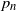, then we prove that the probability of a random binary network being deficiency zero converges to 1 if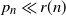as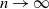, and converges to 0 if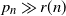as, where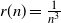.

]]>
https://dx.doi.org/10.1017/jpr.2021.65?rft_dat=source%3Ddrssdoi:10.1017/jpr.2021.65Prevalence of deficiency-zero reaction networks in an Erdös–Rényi framework38439810.1017/jpr.2021.65https://dx.doi.org/10.1017/jpr.2021.65?rft_dat=source%3Ddrss2022-01-28Journal of Applied Probability2022-01-28Anderson, David F.Nguyen, Tung D.
<![CDATA[Large-deviation results for triangular arrays of semiexponential random variables]]>

Asymptotics deviation probabilities of the sum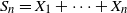of independent and identically distributed real-valued random variables have been extensively investigated, in particular when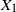is not exponentially integrable. For instance, Nagaev (1969a, 1969b) formulated exact asymptotics results for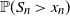with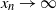whenhas a semiexponential distribution. In the same setting, Brosset et al. (2020) derived deviation results at logarithmic scale with shorter proofs relying on classical tools of large-deviation theory and making the rate function at the transition explicit. In this paper we exhibit the same asymptotic behavior for triangular arrays of semiexponentially distributed random variables.

]]>
https://dx.doi.org/10.1017/jpr.2021.58?rft_dat=source%3Ddrssdoi:10.1017/jpr.2021.58Large-deviation results for triangular arrays of semiexponential random variables39942010.1017/jpr.2021.58https://dx.doi.org/10.1017/jpr.2021.58?rft_dat=source%3Ddrss2022-02-08Journal of Applied Probability2022-02-08Klein, ThierryLagnoux, AgnèsPetit, Pierre
<![CDATA[Renewal theory for iterated perturbed random walks on a general branching process tree: intermediate generations]]>

An iterated perturbed random walk is a sequence of point processes defined by the birth times of individuals in subsequent generations of a general branching process provided that the birth times of the first generation individuals are given by a perturbed random walk. We prove counterparts of the classical renewal-theoretic results (the elementary renewal theorem, Blackwell’s theorem, and the key renewal theorem) for the number of jth-generation individuals with birth times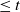, when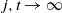and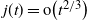. According to our terminology, such generations form a subset of the set of intermediate generations.

]]>
https://dx.doi.org/10.1017/jpr.2021.60?rft_dat=source%3Ddrssdoi:10.1017/jpr.2021.60Renewal theory for iterated perturbed random walks on a general branching process tree: intermediate generations42144610.1017/jpr.2021.60https://dx.doi.org/10.1017/jpr.2021.60?rft_dat=source%3Ddrss2022-02-18Journal of Applied Probability2022-02-18Bohun, VladyslavIksanov, AlexanderMarynych, AlexanderRashytov, Bohdan
<![CDATA[Convergence rate of the EM algorithm for SDEs with low regular drifts]]>

In this paper we employ a Gaussian-type heat kernel estimate to establish Krylov’s estimate and Khasminskii’s estimate for the Euler–Maruyama (EM) algorithm. For applications, by taking Zvonkin’s transformation into account, we investigate the convergence rate of the EM algorithm for a class of multidimensional stochastic differential equations (SDEs) with low regular drifts, which need not be piecewise Lipschitz.

]]>
https://dx.doi.org/10.1017/jpr.2021.56?rft_dat=source%3Ddrssdoi:10.1017/jpr.2021.56Convergence rate of the EM algorithm for SDEs with low regular drifts44747010.1017/jpr.2021.56https://dx.doi.org/10.1017/jpr.2021.56?rft_dat=source%3Ddrss2022-02-14Journal of Applied Probability2022-02-14Bao, JianhaiHuang, XingZhang, Shao-Qin
<![CDATA[On large-deviation probabilities for the empirical distribution of branching random walks with heavy tails]]>

Given a branching random walk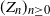on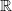, let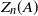be the number of particles located in interval A at generation n. It is well known that under some mild conditions,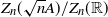converges almost surely to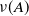as, whereis the standard Gaussian measure. We investigate its large-deviation probabilities under the condition that the step size or offspring law has a heavy tail, i.e. a decay rate of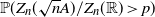as, where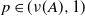. Our results complete those in Chen and He (2019) and Louidor and Perkins (2015).

]]>
https://dx.doi.org/10.1017/jpr.2021.66?rft_dat=source%3Ddrssdoi:10.1017/jpr.2021.66On large-deviation probabilities for the empirical distribution of branching random walks with heavy tails47149410.1017/jpr.2021.66https://dx.doi.org/10.1017/jpr.2021.66?rft_dat=source%3Ddrss2022-03-24Journal of Applied Probability2022-03-24Zhang, Shuxiong
<![CDATA[An averaging process on hypergraphs]]>

Consider the following iterated process on a hypergraph H. Each vertex v starts with some initial weight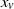. At each step, uniformly at random select an edge e in H, and for each vertex v in e replace the weight of v by the average value of the vertex weights over all vertices in e. This is a generalization of an interactive process on graphs which was first introduced by Aldous and Lanoue. In this paper we use the eigenvalues of a Laplacian for hypergraphs to bound the rate of convergence for this iterated averaging process.

]]>
<![CDATA[On the asymptotic behavior of the Diaconis–Freedman chain in a multi-dimensional simplex]]>

We give a setting of the Diaconis–Freedman chain in a multi-dimensional simplex and consider its asymptotic behavior. By using techniques from random iterated function theory and quasi-compact operator theory, we first give some sufficient conditions which ensure the existence and uniqueness of an invariant probability measure and, in particular cases, explicit formulas for the invariant probability density. Moreover, we completely classify all behaviors of this chain in dimension two. Some other settings of the chain are also discussed.

]]>
https://dx.doi.org/10.1017/jpr.2021.64?rft_dat=source%3Ddrssdoi:10.1017/jpr.2021.64On the asymptotic behavior of the Diaconis–Freedman chain in a multi-dimensional simplex50552610.1017/jpr.2021.64https://dx.doi.org/10.1017/jpr.2021.64?rft_dat=source%3Ddrss2022-01-28Journal of Applied Probability2022-01-28Peigné, MarcTran, Tat Dat
<![CDATA[Optimal discounted drawdowns in a diffusion approximation under proportional reinsurance]]>

A diffusion approximation to a risk process under dynamic proportional reinsurance is considered. The goal is to minimise the discounted time in drawdown; that is, the time where the distance of the present surplus to the running maximum is larger than a given level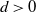. We calculate the value function and determine the optimal reinsurance strategy. We conclude that the drawdown measure stabilises process paths but has a drawback as it also prevents surpassing the initial maximum. That is, the insurer is, under the optimal strategy, not interested in any more profits. We therefore suggest using optimisation criteria that do not avoid future profits.

]]>
https://dx.doi.org/10.1017/jpr.2021.68?rft_dat=source%3Ddrssdoi:10.1017/jpr.2021.68Optimal discounted drawdowns in a diffusion approximation under proportional reinsurance52754010.1017/jpr.2021.68https://dx.doi.org/10.1017/jpr.2021.68?rft_dat=source%3Ddrss2022-03-30Journal of Applied Probability2022-03-30Brinker, Leonie ViolettaSchmidli, Hanspeter
<![CDATA[An explicit Dobrushin uniqueness region for Gibbs point processes with repulsive interactions]]>

We present a uniqueness result for Gibbs point processes with interactions that come from a non-negative pair potential; in particular, we provide an explicit uniqueness region in terms of activity z and inverse temperature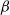. The technique used relies on applying to the continuous setting the classical Dobrushin criterion. We also present a comparison to the two other uniqueness methods of cluster expansion and disagreement percolation, which can also be applied for this type of interaction.

]]>
https://dx.doi.org/10.1017/jpr.2021.70?rft_dat=source%3Ddrssdoi:10.1017/jpr.2021.70An explicit Dobrushin uniqueness region for Gibbs point processes with repulsive interactions54155510.1017/jpr.2021.70https://dx.doi.org/10.1017/jpr.2021.70?rft_dat=source%3Ddrss2022-03-30Journal of Applied Probability2022-03-30Houdebert, PierreZass, Alexander
<![CDATA[Ruin probabilities with investments: smoothness, inegro-differential and ordinary differential equations, asymptotic behavior]]>

This study deals with the ruin problem when an insurance company having two business branches, life insurance and non-life insurance, invests its reserves in a risky asset with the price dynamics given by a geometric Brownian motion. We prove a result on the smoothness of the ruin probability as a function of the initial capital, and obtain for it an integro-differential equation understood in the classical sense. For the case of exponentially distributed jumps we show that the survival (as well as the ruin) probability is a solution of an ordinary differential equation of the fourth order. Asymptotic analysis of the latter leads to the conclusion that the ruin probability decays to zero in the same way as in the already studied cases of models with one-sided jumps.

]]>
https://dx.doi.org/10.1017/jpr.2021.74?rft_dat=source%3Ddrssdoi:10.1017/jpr.2021.74Ruin probabilities with investments: smoothness, inegro-differential and ordinary differential equations, asymptotic behavior55657010.1017/jpr.2021.74https://dx.doi.org/10.1017/jpr.2021.74?rft_dat=source%3Ddrss2022-06-24Journal of Applied Probability2022-06-24Kabanov, YuriPukhlyakov, Nikita
<![CDATA[On the Sn/n problem]]>

The Chow–Robbins game is a classical, still partly unsolved, stopping problem introduced by Chow and Robbins in 1965. You repeatedly toss a fair coin. After each toss, you decide whether you take the fraction of heads up to now as a payoff, otherwise you continue. As a more general stopping problem this reads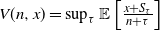, where S is a random walk. We give a tight upper bound for V when S has sub-Gaussian increments by using the analogous time-continuous problem with a standard Brownian motion as the driving process. For the Chow–Robbins game we also give a tight lower bound and use these to calculate, on the integers, the complete continuation and the stopping set of the problem for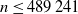.

]]>